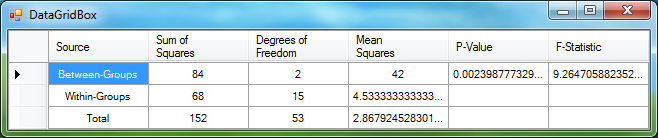﻿ OneWayAnova Class# OneWayAnova Class

One-way Analysis of Variance (ANOVA).Inheritance Hierarchy
SystemObject
Accord.Statistics.TestingOneWayAnova

Namespace:  Accord.Statistics.Testing
Assembly:  Accord.Statistics (in Accord.Statistics.dll) Version: 3.8.0Syntax
```[SerializableAttribute]
public class OneWayAnova : IAnova```

The OneWayAnova type exposes the following members.Constructors
NameDescriptionOneWayAnova(Double)
Creates a new one-way ANOVA test.OneWayAnova(Double, Int32)
Creates a new one-way ANOVA test.
TopProperties
NameDescriptionFTest
Gets the F-Test produced by this one-way ANOVA.Table
Gets the ANOVA results in the form of a table.
TopMethods
NameDescriptionEquals
Determines whether the specified object is equal to the current object.
(Inherited from Object.)Finalize
Allows an object to try to free resources and perform other cleanup operations before it is reclaimed by garbage collection.
(Inherited from Object.)GetHashCode
Serves as the default hash function.
(Inherited from Object.)GetType
Gets the Type of the current instance.
(Inherited from Object.)MemberwiseClone
Creates a shallow copy of the current Object.
(Inherited from Object.)ToString
Returns a string that represents the current object.
(Inherited from Object.)
TopExtension Methods
NameDescriptionHasMethod
Checks whether an object implements a method with the given name.
(Defined by ExtensionMethods.)IsEqual
Compares two objects for equality, performing an elementwise comparison if the elements are vectors or matrices.
(Defined by Matrix.)To(Type)Overloaded.
Converts an object into another type, irrespective of whether the conversion can be done at compile time or not. This can be used to convert generic types to numeric types during runtime.
(Defined by ExtensionMethods.)ToTOverloaded.
Converts an object into another type, irrespective of whether the conversion can be done at compile time or not. This can be used to convert generic types to numeric types during runtime.
(Defined by ExtensionMethods.)
TopRemarks

The one-way ANOVA is a way to test for the equality of three or more means at the same time by using variances. In its simplest form ANOVA provides a statistical test of whether or not the means of several groups are all equal, and therefore generalizes t-test to more than two groups.

References:Examples

The following is the same example given in Wikipedia's page for the F-Test . Suppose one would like to test the effect of three levels of a fertilizer on plant growth.

To achieve this goal, an experimenter has divided a set of 18 plants on three groups, 6 plants each. Each group has received different levels of the fertilizer under question.

After some months, the experimenter registers the growth for each plant:

```double[][] samples =
{
new double[] {  6,  8,  4,  5,  3,  4 }, // records for the first group
new double[] {  8, 12,  9, 11,  6,  8 }, // records for the second group
new double[] { 13,  9, 11,  8,  7, 12 }, // records for the third group
};```

Now, he would like to test whether the different fertilizer levels has indeed caused any effect in plant growth. In other words, he would like to test if the three groups are indeed significantly different.

```// To do it, he runs an ANOVA test:
OneWayAnova anova = new OneWayAnova(samples);```

After the Anova object has been created, one can display its findings in the form of a standard ANOVA table by binding anova.Table to a DataGridView or any other display object supporting data binding. To illustrate, we could use Accord.NET's DataGridBox to inspect the table's contents.

`DataGridBox.Show(anova.Table);`

Result in:The p-level for the analysis is about 0.002, meaning the test is significant at the 5% significance level. The experimenter would thus reject the null hypothesis, concluding there is a strong evidence that the three groups are indeed different. Assuming the experiment was correctly controlled, this would be an indication that the fertilizer does indeed affect plant growth.

 http://en.wikipedia.org/wiki/F_testSee Also